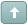Welcome Guest! To enable all features please Login or Register.Error

 Previous Topic Next Topic
 KTP2 #1 Posted : Wednesday, November 12, 2008 4:28:01 PM(UTC)Rank: Advanced MemberGroups: Registered, Registered Users, SubscribersJoined: 2/2/2007(UTC)Posts: 367Was thanked: 1 time(s) in 1 post(s) In his article "Squelch Those Whipsaws" John Ehlers introduces the Squelch Control indicator as a paintbar. To create this in MetaStock 6.52 or higher select Indicator Builder from the Tools menu and enter the following new formulas. Each formula must be created separately and must be named exactly as it appears below. The formulas can also be downloaded from http://www.equis.com/customer/support/formulas/. Note that all of the following formulas are the same formulas referenced from his article in the May 2000 Traders Tip, with the exception of the Expert Advisor highlight. If you have downloaded and installed the May 2000 formulas you will be asked to overwrite the existing formulas when installing these formulas. If you do not wish to overwrite them do not download the formula file, instead refer to the instructions below for creating only the Expert Advisor formula. If you are using MetaStock version 7 or higher follow these instructions:
• Click here to download the self extracting formula file to a temp folder
• Double click on the downloaded file to start the importing process
• After the import has finished locate the formulas in MetaStock If you are using MetaStock version 6.52 use these instructions:
• Double-click on the downloaded file to extract the MS65FORM.DTA and MS65EXPT.DTA files to a temp folder.
• Run MetaStock.
• Choose Expert Advisor from the Tools menu.
• Click the Organizer button to launch the Formula Organizer Wizard.
• Follow the on-screen instructions.
• After the formulas have been imported simply attach the "Squelch Threshold" expert to your chart. Name: Hilbert cycle period - 1avalue1:=((H+L)/2) - Ref(((H+L)/2),-6);value2:= Ref(value1,-3);value3:=0.75*(value1-Ref(value1,-6)) + 0.25*(Ref(value1,-2)-Ref(value1,-4)); inphase:= 0.33 * value2 + (0.67 * PREV);quad:= 0.2 * value3 + ( 0.8 * PREV); p1:=Atan(Abs(quad+Ref(quad,-1)),Abs(inphase+Ref(inphase,-1))); phase:=If(inphase<0 AND quad>0, 180-p1,If(inphase<0 AND quad<0, 180+p1,If(inphase>0 AND quad<0, 360-p1,p1))); dp:=If(Ref(phase,-1)<90 AND phase>270, 360+Ref(phase,-1)-phase,Ref(phase,-1)-phase);dp2:=If(dp < 1, 1,If(dp > 60, 60, dp)); dp2 Name: H cycle count 1avalue:= Fml("Hilbert cycle period - 1a");If(Sum(value,6)>=360 AND Sum(value,5)<360 ,6,0) +If(Sum(value,7)>=360 AND Sum(value,6)<360 ,7,0) +If(Sum(value,8)>=360 AND Sum(value,7)<360 ,8,0) +If(Sum(value,9)>=360 AND Sum(value,8)<360 ,9,0) +If(Sum(value,10)>=360 AND Sum(value,9)<360 ,10,0) +If(Sum(value,11)>=360 AND Sum(value,10)<360 ,11,0) +If(Sum(value,12)>=360 AND Sum(value,11)<360 ,12,0) +If(Sum(value,13)>=360 AND Sum(value,12)<360 ,13,0) +If(Sum(value,14)>=360 AND Sum(value,13)<360 ,14,0) +If(Sum(value,15)>=360 AND Sum(value,14)<360 ,15,0) Name: H cycle count 2avalue:= Fml("Hilbert cycle period - 1a");If(Sum(value,16)>=360 AND Sum(value,15)<360 ,16,0) +If(Sum(value,17)>=360 AND Sum(value,16)<360 ,17,0) +If(Sum(value,18)>=360 AND Sum(value,17)<360 ,18,0) +If(Sum(value,19)>=360 AND Sum(value,18)<360 ,19,0) +If(Sum(value,20)>=360 AND Sum(value,19)<360 ,20,0) +If(Sum(value,21)>=360 AND Sum(value,20)<360 ,21,0) +If(Sum(value,22)>=360 AND Sum(value,21)<360 ,22,0) +If(Sum(value,23)>=360 AND Sum(value,22)<360 ,23,0) +If(Sum(value,24)>=360 AND Sum(value,23)<360 ,24,0) +If(Sum(value,25)>=360 AND Sum(value,24)<360 ,25,0) Name: H cycle count 3avalue:= Fml("Hilbert cycle period - 1a");If(Sum(value,26)>=360 AND Sum(value,25)<360 ,26,0) +If(Sum(value,27)>=360 AND Sum(value,26)<360 ,27,0) +If(Sum(value,28)>=360 AND Sum(value,27)<360 ,28,0) +If(Sum(value,29)>=360 AND Sum(value,28)<360 ,29,0) +If(Sum(value,30)>=360 AND Sum(value,29)<360 ,30,0) +If(Sum(value,31)>=360 AND Sum(value,30)<360 ,31,0) +If(Sum(value,32)>=360 AND Sum(value,31)<360 ,32,0) +If(Sum(value,33)>=360 AND Sum(value,32)<360 ,33,0) +If(Sum(value,34)>=360 AND Sum(value,33)<360 ,34,0) +If(Sum(value,35)>=360 AND Sum(value,34)<360 ,35,0) Name: Hilbert cycle period - final-ac1:= Fml( "H cycle count 1a") + Fml( "H cycle count 2a") + Fml( "H cycle count 3a") ;c2:=If(c1=0,PREV,c1); (0.25*c2) + (0.75*PREV) To create the indicator as a highlight, select Expert Advisor from the Tools menu. Click New, enter "Hilbert Squelch Indicator" for the name, and select the Highlights page. Click New and enter the following formula: Name: Squelch ThresholdSquelch:=20;Fml("Hilbert cycle period - final-a")
•  Wanna join the discussion?! Login to your Discussions forum accountor Register a new forum account.Users browsing this topic
Forum Jump
You cannot post new topics in this forum.
You cannot reply to topics in this forum.
You cannot delete your posts in this forum.
You cannot edit your posts in this forum.
You cannot create polls in this forum.
You cannot vote in polls in this forum.